The Sundial Primer created by Carl SabanskiThe Sundial Primer Index
 Declination of a Wall Declination of a wall (d, DEC): the angle, measured in a horizontal plane, that a wall's perpendicular makes with due south (i.e. a wall facing S has d=0°). Walls declining westward have positive declinations, those eastward, negative. This is not a universal convention and some authors define the angle with respect to the nearest cardinal point of the compass.Declination of the sun (δ. DELTA, DEC): the angular distance of the Sun above or below the celestial equator. Its value follows an annual sine wave like curve, varying from 0° at the equinoxes and ±23.4° (approx.) at the solstices. It has positive values when the sun is above the celestial equator (summer in the Northern hemisphere) and negative when below.Azimuth of the sun (A, AZ): the angle of the sun, measured in the horizontal plane and from true south.  Angles to the west are positive, those to the east negative. Thus due west is 90°, north is ±180°, east -90°. The design of a vertical sundial that does not face one of the cardinal directions north, south, east or west requires that you determine the declination of the wall that it will be mounted on. To do this you must determine two things: the direction of the sun relative to the wall  - θ the direction of the sun relative to true south, i.e. its azimuth - A To determine the direction of the sun relative to the wall the device shown in Figure 1 must be constructed. It consists of a board with a long nail attached near the top at the centre of the board. If you cannot draw on the board, firmly attach a large sheet of paper to the surface before installing the nail. A vertical line is drawn from the nail down to the bottom of the paper. A plumb line is attached to the nail consisting of a string and weight at the bottom end. Measure the length of the nail as accurately as possible. Use as long a nail as possible and ensure that it is perpendicular to the surface of the board. Securely mount the entire assembly to the surface of the wall rotating it so that the plumb line falls along the line drawn on the paper. Make sure that the board is not twisted to the top or bottom. This will ensure that the nail is perpendicular to the vertical line of the wall. It must also be aligned as straight as possible with the wall in the horizontal direction.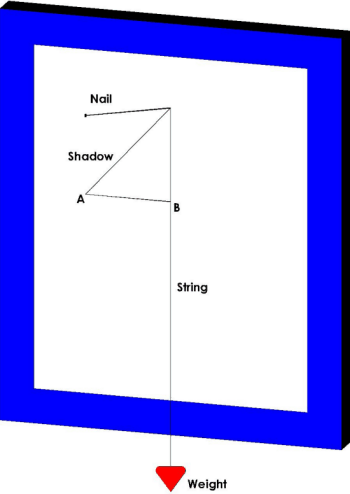Figure 1: Determining the Direction of the Sun Relative to the Wall The next step requires that you have a watch that is set as accurately as possible. At a given time mark the position of the end of the nail's shadow on the paper. Record the time and date. This step should be done 3 or 4 times at half hour intervals. Once this is complete remove the device. Draw a line AB at right angles to the vertical line to a shadow point marked on the paper. A line is drawn for each of the other marked points. Measure these distances as accurately as possible. The direction of the sun relative to the wall, θ, can be determined as follows: θ = arctan( AB / Nail Length)° Determine this value for each of the measurements taken. The azimuth of the sun must now be determined for each of the measurements taken. The sun's azimuth is given by the following equation: A = arctan[ sin(h) / {sinø * cos(h) - cosø * tanδ} ] ° where: ø: is the latitude δ: is the sun's declination h: is the hour angle, in degrees, given by h = (T24 - 12) x 15° where T24 is the time in 24-hour clock notation (hours after midnight) in decimal hours. Note that the clock time recorded must first be converted to Local Apparent Time before calculating h. Determining the sun's declination by calculation is not that easy and then you must perform the calculation to determine the sun's azimuth A. You may pursue this further if you wish but a simple method of determining the sun's azimuth is to use The Dialist's Companion. This program allows you to set any location, date and time. To accomplish this, the date of the measurements would first be entered. Then the "Pinawa Central" time would be entered as one of the times recorded and the "End" key selected to freeze the screen. As long as the "End"  key is not selected again the "Pinawa Central" time can be adjusted to any time and the clock will remain frozen. The sun's azimuth is recorded from the "Azimuth" display. This is done for each of the recorded times.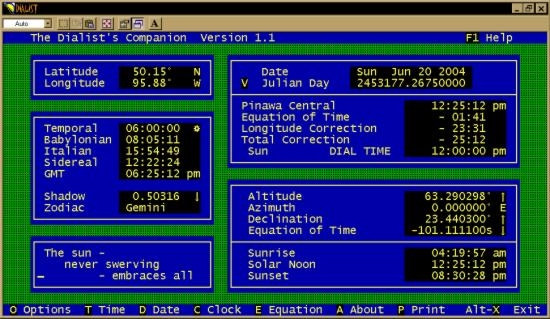Figure 2: Determining the sun's azimuth using "The Dialist's Companion". You should now have two sets of values for each of the times that a measurement was takes. One is the direction of the sun relative to the wall and the other is the sun's azimuth. The declination of the wall can now be determined using the following rules. The measurements were taken prior to local apparent noon. The sun was to the right of the wall.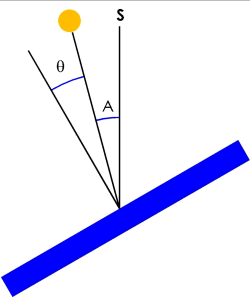The wall declines to the east by: A + θ. The sun was to the left of the wall and A is greater than θ.The wall declines to the east by: A - θ. The sun was to the left of the wall and θ is greater than A.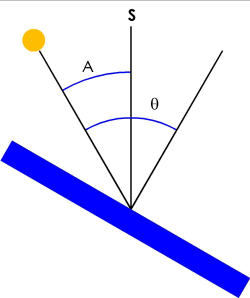The wall declines to the west by: θ - A. The measurements were taken after local apparent noon. The sun was to the left of the wall.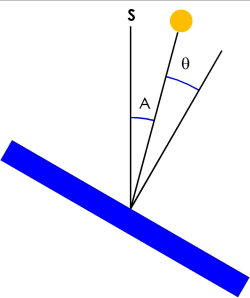The wall declines to the west by: A + θ . The sun was to the right of the wall and A is greater than θ.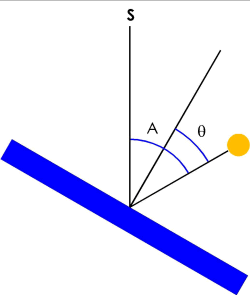The wall declines to the west by: A - θ. The sun was to the right of the wall and θ is greater than A.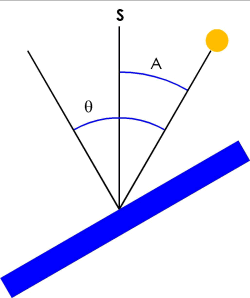The wall declines to the east by: θ - A. This calculation of the wall's declination, d, would be done for each recorded measurement. These values are likely to be slightly different due to errors in the measurements and calculations. If one value is significantly different than the others redo the calculation. By averaging these calculated values, the error in the final wall's declination will be reduced. The average value is determined as follows: dav = {value1 + value2 + value3 + ....... + value(n)} / n where n = the total number of measurements taken with the resulting calculated values value1, value2, etc.